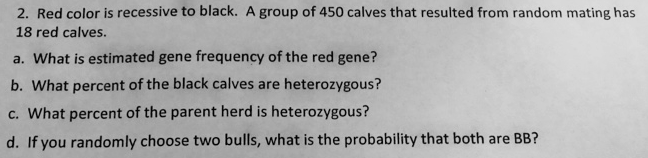# Problem: Red color is recessive to black. A group of 450 calves that resulted from random mating has 18 red calves. A. What is estimated gene frequency of the red gene?B. What percent of the black calves are heterozygous?C. What percent of the parent herd is heterozygous?D. If you randomly choose two bulls, what is the probability that both are BB?

###### FREE Expert Solution

Assuming that the population is large enough to follow Hardy Weinberg equilibrium, p2 + 2pq + q2 = 1, where p and q are the expected frequency of the dominant and recessive allele, respectively, and p2 and q2 are the expected frequency of the homozygous dominant and homozygous recessive genotypes, respectively, we can use the given data to arrive at the following estimates:###### Problem Details

Red color is recessive to black. A group of 450 calves that resulted from random mating has 18 red calves.

A. What is estimated gene frequency of the red gene?

B. What percent of the black calves are heterozygous?

C. What percent of the parent herd is heterozygous?

D. If you randomly choose two bulls, what is the probability that both are BB?